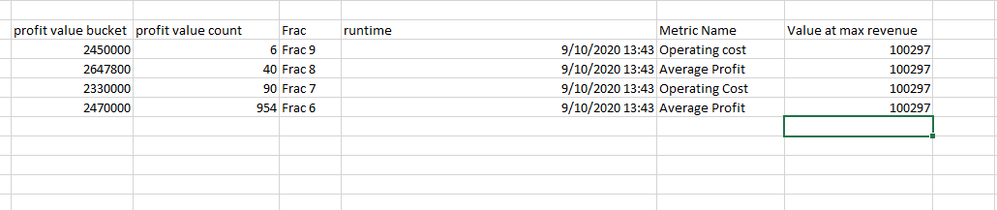cancel
Showing results for
Did you mean:Anonymous
Not applicable

## Normal Distribution Curve

Hi,

I created normal distribution curve from Joseph Romero videos but the values don't change if I try to change the date.

Below are the formulas I used.

Please let me know how I can filter with date values

f(x) =
EXP((VALUES('Normal Distribution'[X])-[Mean new])^2/(2*[STDEV new]^2)*-1)/(SQRT(2*PI())*[STDEV new])
Normal Distribution =
VAR MinValue = Floor([-2 sigma new],1)
VAR MaxValue = CEILING([+2 sigma new],1)
RETURN
SELECTCOLUMNS(CALENDAR(MinValue,MaxValue),"X",INT([Date]))
Z = ('Normal Distribution'[X]-[Mean new]/[STDEV new])
4 REPLIES 4Community Support

Hi  @Anonymous  ,

Do the suggestions from engineers make sense? If so, kindly mark the proper reply as a solution to help others having the similar issue and close the case. If not, let me know and I'll try to help you further.

Best regards

AmySuper User

@Anonymous Can you post sample data or PBIX link? Not quite sure about your use of CALENDAR there. I also don't like VALUES.

Become an expert!: Enterprise DNA
External Tools: MSHGQM
Latest book!:
Mastering Power BI 2nd EditionDAX is easy, CALCULATE makes DAX hard...Anonymous
Not applicable

@Greg_Deckler  Here's the sample data. I used this table to calculate mean and standard deviation.

I want to use run time dates to filter the normal distribution curve.Community Support

Hi @Anonymous ,

You may use measure instead of calculated column to create the formula. You couldn't have a calculated column interact with a slicer since columns are only calculated at data load/refresh.

Best Regards,

Amy

Community Support Team _ Amy

If this post helps, then please consider Accept it as the solution to help the other members find it more quickly.